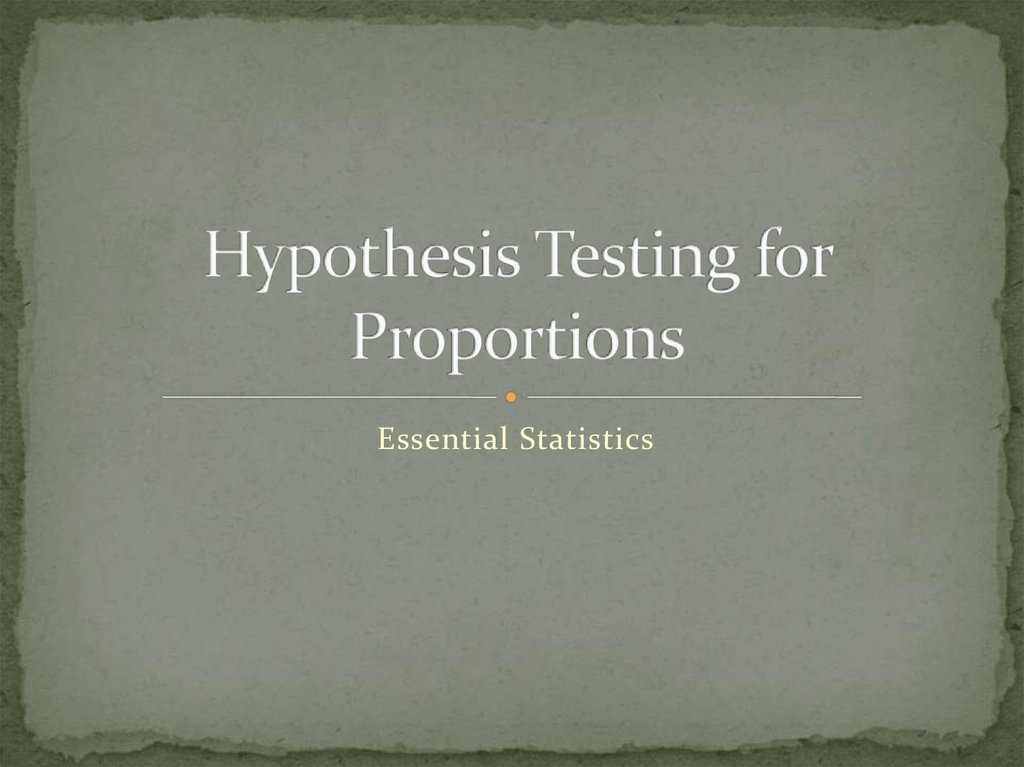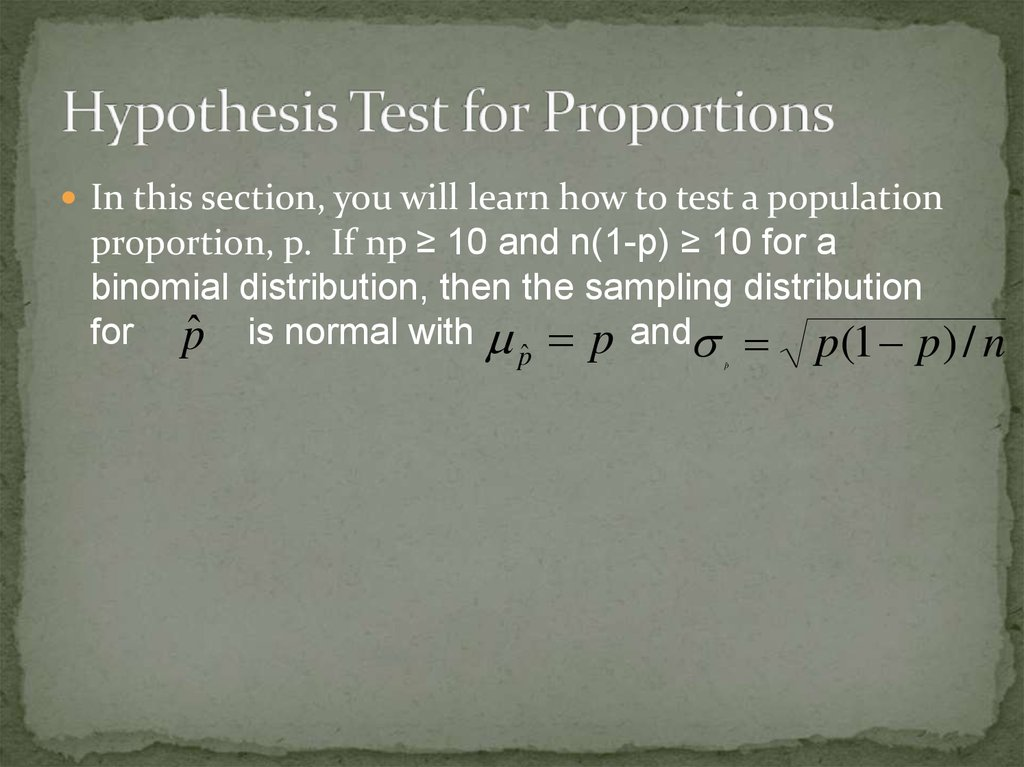# Hypothesis testing for proportions. Essential statistics

## 1. Hypothesis Testing for Proportions

Essential Statistics

## 2. Hypothesis Test for Proportions

In this section, you will learn how to test a population
proportion, p. If np ≥ 10 and n(1-p) ≥ 10 for a
binomial distribution, then the sampling distribution
for p
ˆ is normal with p and p(1 p) / n

## 3. Steps for doing a hypothesis test

“Since the p-value < (>) a, I reject
1) Assumptions
(fail to reject) the H0. There is (is
not) sufficient evidence to suggest
thathypotheses
Ha (in context).”
2) Write
& define parameter
3) Calculate the test statistic & p-value
4) Write a statement in the context of the
problem.

## 4. What is the p-value

The P-Value is the probability of obtaining a test
statistic that is at least as extreme as the one that was
actually observed, assuming the null is true.
p-value < (>) a, I reject (fail to reject) the H0.

## 5. How to calculate the P-value

Under Stat – Tests
Select 1 Prop Z-test
Input p, x, and n
P is claim proportion
X is number of sampling matching claim
N is number sampled
Select correct Alternate Hypothesis
Calculate

Provides you with the z score
P-Value
Sample proportion
Interpret the p-value based off of your Confidence
interval

## 7. Draw & shade a curve & calculate the p-value:

1) right-tail test
z = 1.6
P-value = .0548
2) two-tail test
z = 2.3
P-value = (.0107)2 = .0214

## 8. What is a

a Represents the remaining percentage of our
confidence interval. 95% confidence interval has a
5% alpha.

## 9. Ex. 1: Hypothesis Test for a Proportion

A medical researcher claims that less than 20% of
American adults are allergic to a medication. In a
random sample of 100 adults, 15% say they have such
an allergy. Test the researcher’s claim at a = 0.01.

## 10. SOLUTION

The products np = 100(0.20)= 20 and nq = 100(0.80) =
80 are both greater than 10. So, you can use the z-test.
The claim is “less than 20% are allergic to a
medication.” So the null and alternative hypothesis
are:
Ho: p = 0.2 and Ha: p < 0.2 (Claim)

## 11. Solution By HAND continued . . .

Because the test is a left-tailed test and the level of
significance is a = 0.01, the critical value is zo = -2.33
and the rejection region is z < -2.33. Using the z-test,
the standardized test statistic is:
pˆ p
z
pq
n
0.15 0.20
1.25
(0.20)(0.80)
100

## 12. SOLUTION Continued . . .

The graph shows the
location of the rejection
region and the standardized
test statistic, z. Because z is
not in the rejection region,
you should decide not to
reject the null hypothesis.
In other words, there is not
enough evidence to support
the claim that less than 20%
of Americans are allergic to
the medication.

## 14. Interpretation

Since the .1056 > .01, I fail to reject the
H0 There is not sufficient evidence to
suggest that 20% of adults are allergic to
medication.

## 15. Ex. 2 Hypothesis Test for a Proportion

Harper’s Index claims that 23% of Americans are in
favor of outlawing cigarettes. You decide to test this
claim and ask a random sample of 200 Americas
whether they are in favor outlawing cigarettes. Of the
200 Americans, 27% are in favor. At a = 0.05, is there
enough evidence to reject the claim?

## 16. SOLUTION:

The products np = 200(0.23) = 45 and nq = 200(0.77) =
154 are both greater than 5. So you can use a z-test.
The claim is “23% of Americans are in favor of
outlawing cigarettes.” So, the null and alternative
hypotheses are:
Ho: p = 0.23 (Claim) and Ha: p 0.23

## 17. SOLUTION continued . . .

Because the test is a two-tailed test, and the level of
significance is a = 0.05.
Z = 1.344
P = .179
Since the .179 > .05, I fail to reject the H0 There is not
sufficient evidence to suggest that more or less than 23%
of Americans are in favor of outlawing cigarette’s.

## 18. SOLUTION Continued . . .

The graph shows the
location of the rejection
regions and the
standardized test statistic, z.
Because z is not in the
rejection region, you should
fail to reject the null
hypothesis. At the 5% level
of significance, there is not
enough evidence to reject
the claim that 23% of
Americans are in favor of
outlawing cigarettes.

## 19. Ex. 3 Hypothesis Test a Proportion

The Pew Research Center claims that more than
55% of American adults regularly watch a network
news broadcast. You decide to test this claim and
ask a random sample of 425 Americans whether
they regularly watch a network news broadcast.
Of the 425 Americans, 255 responded yes. At a =
0.05, is there enough evidence to support the
claim?

## 20. SOLUTION:

The products np = 425(0.55) = 235 and nq =
425(0.45) = 191 are both greater than 5. So you can
use a z-test. The claim is “more than 55% of
Americans watch a network news broadcast.” So,
the null and alternative hypotheses are:
Ho: p = 0.55 and Ha: p > 0.55 (Claim)

## 21. SOLUTION continued . . .

Because the test is a right-tailed test, and the level of
significance is a = 0.05.
Z = 2.072
P-value = .019
Since the 0.019 < .05, I reject the H0. There is sufficient
evidence to suggest that 20% of adults are allergic to
medication.

## 22. SOLUTION Continued . . .

The graph shows the
location of the rejection
region and the standardized
test statistic, z. Because z is
in the rejection region, you
should decide to There is
enough evidence at the 5%
level of significance, to
support the claim that 55%